# 0x00 简介

Android apk很容易通过逆向工程进行反编译，从而是其代码完全暴露给攻击者，使apk面临破解，软件逻辑修改，插入恶意代码，替换广告商ID等风险。我们可以采用以下方法对apk进行保护.

# 0x01 混淆保护

1. 通过对代码类名，函数名做替换来实现代码混淆保护
2. 简单的逻辑分支混淆

• Proguard
• DashO
• Dexguard
• DexProtector
• ApkProtect
• Shield4j
• Stringer
• Allitori

``````#!bash
Java Code(.java) -> Java Bytecode(.class) -> Dalvik Bytecode(classes.dex)
``````

1. 无法真正保护代码不被反编译；
2. 在应对动态调试逆向分析上无效；
3. 通过验证本地签名的机制很容易被绕过。

http://www.jianshu.com/p/0c23e0a886f4

# 0x02 二次打包防护

### 2.1 Apk签名校验

``````#!java
public class getSign {
public static int getSignature(PackageManager pm , String packageName){
PackageInfo pi = null;
int sig = 0;
Signature[]s = null;
try{
pi = pm.getPackageInfo(packageName, PackageManager.GET_SIGNATURES);
s = pi.signatures;
sig = s.hashCode();//s是签名证书的公钥，此处获取hashcode方便对比
}catch(Exception e){
handleException();
}
return sig;
}
}
``````

``````#!java
pm = this.getPackageManager();
int s = getSign.getSignature(pm, "com.hik.getsinature");
if(s != ORIGNAL_SGIN_HASHCODE){//对比当前和预埋签名的hashcode是否一致
System.exit(1);//不一致则强制程序退出
}
``````

### 2.2 Dex文件校验

1. 读取应用安装目录下`/data/app/xxx.apk`中的classes.dex文件并计算其哈希值，将该值与软件发布时的classes.dex哈希值做比较来判断客户端是否被篡改。
2. 读取应用安装目录下`/data/app/xxx.apk`中的META-INF目录下的MANIFEST.MF文件，该文件详细记录了apk包中所有文件的哈希值，因此可以读取该文件获取到classes.dex文件对应的哈希值，将该值与软件发布时的classes.dex哈希值做比较就可以判断客户端是否被篡改。

``````#!java
private boolean checkcrc(){
boolean checkResult = false;
long crc = Long.parseLong(getString(R.string.crc));//获取字符资源中预埋的crc值
ZipFile zf;
try{
String path = getApplicationContext().getPackageCodePath();//获取apk安装路径
zf = new ZipFile(path);//将apk封装成zip对象
ZipEntry ze = zf.getEntry("classes.dex");//获取apk中的classes.dex
long CurrentCRC = ze.getCrc();//计算当前应用classes.dex的crc值
if(CurrentCRC != crc){//crc值对比
checkResult = true;
}
}catch(IOException e){
handleError();
checkResult = false;
}
return checkResult;
}
``````

# 0x03 SO保护

Android so通过C/C++代码来实现，相对于Java代码来说其反编译难度要大很多，但对于经验丰富的破解者来说，仍然是很容易的事。应用的关键性功能或算法，都会在so中实现，如果so被逆向，应用的关键性代码和算法都将会暴露。对于so的保护，可以才有编译器优化技术、剥离二进制文件等方式，还可以使用开源的so加固壳upx进行加固。

UPX对文件进行加壳时会把软件版本等相关信息写入壳内，攻击者可以通过静态反汇编可查看到这些壳信息，进而寻找对应的脱壳机进行脱壳，使得攻击难度降低。所以我们必须在UPX源码中删除这些信息，重新编译后再进行加壳，步骤如下：

1. 使用原始版本对文件进行加壳。
2. 使用IDA反汇编加壳文件，在反汇编文件的上下文中查找UPX壳特征字符串。
3. 在UPX源码中查找这些特征字符串，并一一删除。

https://www.nowsecure.com/resources/secure-mobile-development/coding-practices/code-complexity-and-obfuscation/

# 0x04 资源文件保护

1. 通过资源定位代码，方便应用破解 反编译apk获得源码，通过资源文件或者关键字符串的ID定位到关键代码位置，为逆向破解应用程序提供方便.
2. 替换资源文件，盗版应用 “if you can see something, you can copy it”。Android应用程序中的资源，比如图片和音频文件，容易被复制和窃取。

# 0x05 反调试技术

### 5.2 Trace检查

a.父进程检测

``````#!cpp
#include <stdio.h>
#include <string.h>

int main(int argc, char *argv[]) {
char buf0, buf1;
FILE* fin;

snprintf(buf0, 24, "/proc/%d/cmdline", getppid());
fin = fopen(buf0, "r");
fgets(buf1, 128, fin);
fclose(fin);

if(!strcmp(buf1, "gdb")) {
printf("Debugger detected");
return 1;
}
printf("All good");
return 0;
}
``````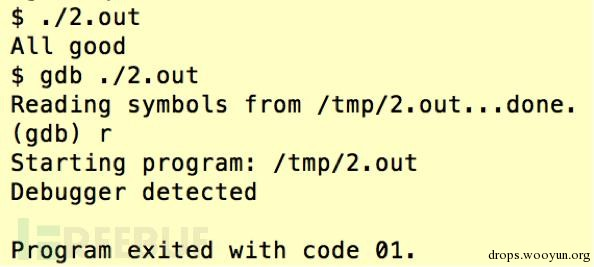b.当前运行进程检测

``````#!java
pid_t GetPidByName(const charchar *as_name) {
DIR *pdir = NULL;
struct dirent *pde = NULL;
FILEFILE *pf = NULL;
char buff;
pid_t pid;
char szName;
// 遍历/proc目录下所有pid目录
pdir = opendir("/proc");
if (!pdir) {
perror("open /proc fail.\n");
return -1;
}
if ((pde->d_name < '0') || (pde->d_name > '9')) {
continue;
}
sprintf(buff, "/proc/%s/status", pde->d_name);
pf = fopen(buff, "r");
if (pf) {
fgets(buff, sizeof(buff), pf);
fclose(pf);
sscanf(buff, "%*s %s", szName);
pid = atoi(pde->d_name);
if (strcmp(szName, as_name) == 0) {
closedir(pdir);
return pid;
}
}
}
closedir(pdir);
return 0;
}
``````

c.读取进程状态(/proc/pid/status)

State属性值T 表示调试状态，TracerPid 属性值正在调试此进程的pid,在非调试情况下State为S或R, TracerPid等于0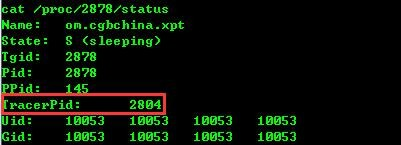``````#!cpp
#include <stdio.h>
#include <string.h>
int main(int argc, char *argv[]) {
int i;
scanf("%d", &i);
char buf1;
FILE* fin;
fin = fopen("/proc/self/status", "r");
int tpid;
const char *needle = "TracerPid:";
size_t nl = strlen(needle);
while(fgets(buf1, 512, fin)) {
if(!strncmp(buf1, needle, nl)) {
sscanf(buf1, "TracerPid: %d", &tpid);
if(tpid != 0) {
printf("Debuggerdetected");
return 1;
}
}
}
fclose(fin);
printf("All good");
return 0;
}
``````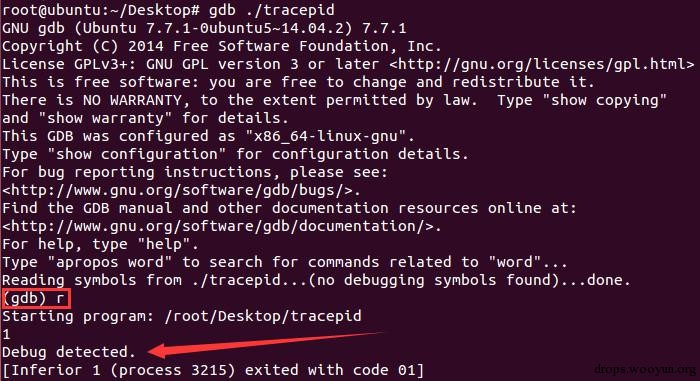d.读取`/proc/%d/wchan`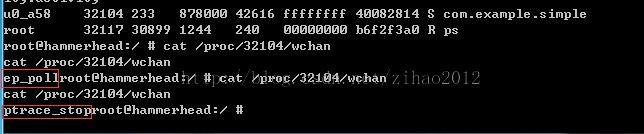``````#!cpp
static int getWchanStatus(int pid)
{
FILEFILE *fp= NULL;
char filename;
char wchaninfo = {0};
int result = WCHAN_ELSE;
char cmd = {0};
sprintf(cmd,"cat /proc/%d/wchan",pid);
LOGANTI("cmd= %s",cmd);
FILEFILE *ptr;
if((ptr=popen(cmd, "r")) != NULL)
{
if(fgets(wchaninfo, 128, ptr) != NULL)
{
LOGANTI("wchaninfo= %s",wchaninfo);
}
}
if(strncasecmp(wchaninfo,"sys_epoll\0",strlen("sys_epoll\0")) == 0)
result = WCHAN_RUNNING;
else if(strncasecmp(wchaninfo,"ptrace_stop\0",strlen("ptrace_stop\0")) == 0)
result = WCHAN_TRACING;
return result;
}
``````

e.ptrace 自身或者fork子进程相互ptrace

``````#!cpp
if (ptrace(PTRACE_TRACEME, 0, 1, 0) < 0) {
printf("DEBUGGING... Bye\n");
return 1;
}
void anti_ptrace(void)
{
pid_t child;
child = fork();
if (child)
wait(NULL);
else {
pid_t parent = getppid();
if (ptrace(PTRACE_ATTACH, parent, 0, 0) < 0)
while(1);
sleep(1);
ptrace(PTRACE_DETACH, parent, 0, 0);
exit(0);
}
}
``````

f.设置程序运行最大时间

``````#!cpp
#include <stdio.h>
#include <signal.h>
#include <stdlib.h>
void alarmHandler(int sig) {
printf("Debugger detected");
exit(1);
}
void__attribute__((constructor))setupSig(void) {
signal(SIGALRM, alarmHandler);
alarm(2);
}
int main(int argc, char *argv[]) {
printf("All good");
return 0;
}
``````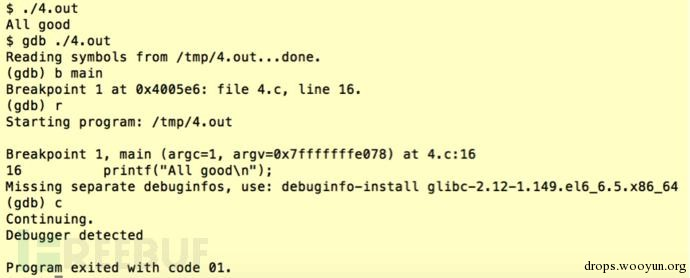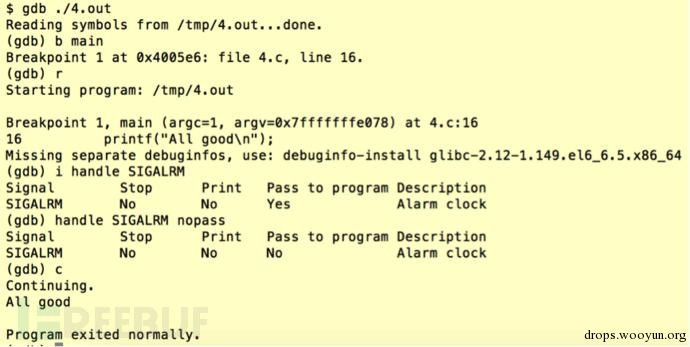g.检查进程打开的filedescriptor

``````#!cpp
#include <stdio.h>
#include <dirent.h>
int main(int argc, char *argv[]) {
struct dirent *dir;
DIR *d = opendir("/proc/self/fd");
if(!strcmp(dir->d_name, "5")) {
printf("Debugger detected");
return 1;
}
}
closedir(d);
printf("All good");
return 0;
}
``````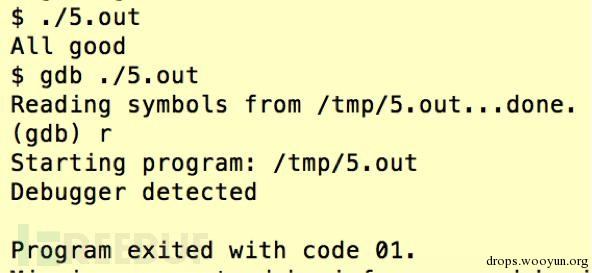h.防止dump

``````#!cpp
void __fastcall anitInotify(int flag)
{
MemorPagemap = flag;
charchar *pagemap = "/proc/%d/pagemap";
charchar *mem = "/proc/%d/mem";
if ( !MemorPagemap )
{
if ( ret >= 0 )
}
if ( MemorPagemap == 1 )
{
if(ret > 0)
}
}
void __fastcall __noreturn inotity_func(const charchar *inotity_file)
{
const charchar *name;[email protected]/* <![CDATA[ */!function(t,e,r,n,c,a,p){try{t=document.currentScript||function(){for(t=document.getElementsByTagName('script'),e=t.length;e--;)if(t[e].getAttribute('data-cfhash'))return t[e]}();if(t&#038;&#038;(c=t.previousSibling)){p=t.parentNode;if(a=c.getAttribute('data-cfemail')){for(e='',r='0x'+a.substr(0,2)|0,n=2;a.length-n;n+=2)e+='%'+('0'+('0x'+a.substr(n,2)^r).toString(16)).slice(-2);p.replaceChild(document.createTextNode(decodeURIComponent(e)),c)}p.removeChild(t)}}catch(u){}}()/* ]]&gt; */
signed int fd;[email protected]/* <![CDATA[ */!function(t,e,r,n,c,a,p){try{t=document.currentScript||function(){for(t=document.getElementsByTagName('script'),e=t.length;e--;)if(t[e].getAttribute('data-cfhash'))return t[e]}();if(t&#038;&#038;(c=t.previousSibling)){p=t.parentNode;if(a=c.getAttribute('data-cfemail')){for(e='',r='0x'+a.substr(0,2)|0,n=2;a.length-n;n+=2)e+='%'+('0'+('0x'+a.substr(n,2)^r).toString(16)).slice(-2);p.replaceChild(document.createTextNode(decodeURIComponent(e)),c)}p.removeChild(t)}}catch(u){}}()/* ]]&gt; */
bool flag;[email protected]/* <![CDATA[ */!function(t,e,r,n,c,a,p){try{t=document.currentScript||function(){for(t=document.getElementsByTagName('script'),e=t.length;e--;)if(t[e].getAttribute('data-cfhash'))return t[e]}();if(t&#038;&#038;(c=t.previousSibling)){p=t.parentNode;if(a=c.getAttribute('data-cfemail')){for(e='',r='0x'+a.substr(0,2)|0,n=2;a.length-n;n+=2)e+='%'+('0'+('0x'+a.substr(n,2)^r).toString(16)).slice(-2);p.replaceChild(document.createTextNode(decodeURIComponent(e)),c)}p.removeChild(t)}}catch(u){}}()/* ]]&gt; */
bool ret;[email protected]/* <![CDATA[ */!function(t,e,r,n,c,a,p){try{t=document.currentScript||function(){for(t=document.getElementsByTagName('script'),e=t.length;e--;)if(t[e].getAttribute('data-cfhash'))return t[e]}();if(t&#038;&#038;(c=t.previousSibling)){p=t.parentNode;if(a=c.getAttribute('data-cfemail')){for(e='',r='0x'+a.substr(0,2)|0,n=2;a.length-n;n+=2)e+='%'+('0'+('0x'+a.substr(n,2)^r).toString(16)).slice(-2);p.replaceChild(document.createTextNode(decodeURIComponent(e)),c)}p.removeChild(t)}}catch(u){}}()/* ]]&gt; */
ssize_t length;[email protected]/* <![CDATA[ */!function(t,e,r,n,c,a,p){try{t=document.currentScript||function(){for(t=document.getElementsByTagName('script'),e=t.length;e--;)if(t[e].getAttribute('data-cfhash'))return t[e]}();if(t&#038;&#038;(c=t.previousSibling)){p=t.parentNode;if(a=c.getAttribute('data-cfemail')){for(e='',r='0x'+a.substr(0,2)|0,n=2;a.length-n;n+=2)e+='%'+('0'+('0x'+a.substr(n,2)^r).toString(16)).slice(-2);p.replaceChild(document.createTextNode(decodeURIComponent(e)),c)}p.removeChild(t)}}catch(u){}}()/* ]]&gt; */
ssize_t i;[email protected]/* <![CDATA[ */!function(t,e,r,n,c,a,p){try{t=document.currentScript||function(){for(t=document.getElementsByTagName('script'),e=t.length;e--;)if(t[e].getAttribute('data-cfhash'))return t[e]}();if(t&#038;&#038;(c=t.previousSibling)){p=t.parentNode;if(a=c.getAttribute('data-cfemail')){for(e='',r='0x'+a.substr(0,2)|0,n=2;a.length-n;n+=2)e+='%'+('0'+('0x'+a.substr(n,2)^r).toString(16)).slice(-2);p.replaceChild(document.createTextNode(decodeURIComponent(e)),c)}p.removeChild(t)}}catch(u){}}()/* ]]&gt; */
fd_set readfds;[email protected]/* <![CDATA[ */!function(t,e,r,n,c,a,p){try{t=document.currentScript||function(){for(t=document.getElementsByTagName('script'),e=t.length;e--;)if(t[e].getAttribute('data-cfhash'))return t[e]}();if(t&#038;&#038;(c=t.previousSibling)){p=t.parentNode;if(a=c.getAttribute('data-cfemail')){for(e='',r='0x'+a.substr(0,2)|0,n=2;a.length-n;n+=2)e+='%'+('0'+('0x'+a.substr(n,2)^r).toString(16)).slice(-2);p.replaceChild(document.createTextNode(decodeURIComponent(e)),c)}p.removeChild(t)}}catch(u){}}()/* ]]&gt; */
char event;[email protected]/* <![CDATA[ */!function(t,e,r,n,c,a,p){try{t=document.currentScript||function(){for(t=document.getElementsByTagName('script'),e=t.length;e--;)if(t[e].getAttribute('data-cfhash'))return t[e]}();if(t&#038;&#038;(c=t.previousSibling)){p=t.parentNode;if(a=c.getAttribute('data-cfemail')){for(e='',r='0x'+a.substr(0,2)|0,n=2;a.length-n;n+=2)e+='%'+('0'+('0x'+a.substr(n,2)^r).toString(16)).slice(-2);p.replaceChild(document.createTextNode(decodeURIComponent(e)),c)}p.removeChild(t)}}catch(u){}}()/* ]]&gt; */
name = inotity_file;
memset(buffer, 0, 0x400u);
fd = inotify_init();
while ( 1 )
{
do
{
}
while ( select(fd + 1, &readfds, 0, 0, 0) <= 0 );
flag = length == 0;
ret = length < 0;
if ( length >= 0 )
{
if ( !ret && !flag )
{
i = 0;
do
{
inotity_kill((int)&event);
i += *(_DWORD *)&event + 16;
}
while ( length > i );
}
}
else
{
while ( *(_DWORD *)_errno() == 4 )
{
flag = length == 0;
ret = length < 0;
if ( length >= 0 )
}
}
}
}
``````

j.设置单步调试陷阱

``````#!cpp
int handler()
{
return bsd_signal(5, 0);
}
int set_SIGTRAP()
{
int result;
bsd_signal(5, (int)handler);
result = raise(5);
return result;
}
``````

http://www.freebuf.com/tools/83509.html

# 0x06 应用加固技术

• 第一代是基于类加载器的方式实现保护；
• 第二代是基于方法替换的方式实现保护；
• 第三代是基于虚拟机指令集的方式实现保护。

1. classes.dex被完整加密，放到APK的资源中
2. 采用动态劫持虚拟机的类载入引擎的技术
3. 虚拟机能够载入并运行加密的classes.dex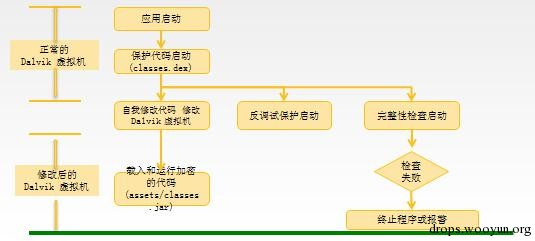1. 将原APK中的所有方法的代码提取出来，单独加密
2. 运行时动态劫持Dalvik虚拟机中解析方法的代码，将解密后的代码交给虚拟机执行引擎

1. 每个方法单独解密，内存中无完整的解密代码
2. 如果某个方法没有执行，不会解密
3. 在内存中dump代码的成本代价很高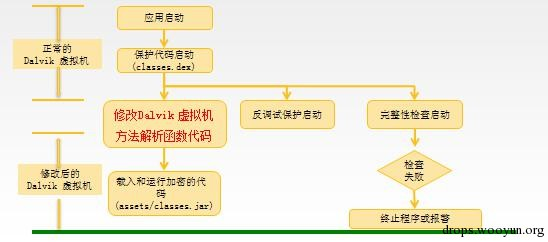1. 将原APK中的所有的代码采用一种自定义的指令格式进行替换
2. 运行时动态劫持Dalvik虚拟机中执行引擎，使用自定义执行引擎执行自定义的代码
3. 类似于PC上的VMProtect采用的技术

1. 具有2.0的所有优点
2. 破解需要破解自定义的指令格式，复杂度非常高